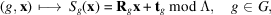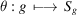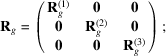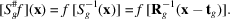International
Tables for
Crystallography
Volume B
Reciprocal space
Edited by U. Shmueli

International Tables for Crystallography (2010). Vol. B, ch. 1.3, pp. 71-72   | 1 | 2 |

## Section 1.3.4.2.2.4. Crystallographic group action in real space

G. Bricognea

aGlobal Phasing Ltd, Sheraton House, Suites 14–16, Castle Park, Cambridge CB3 0AX, England, and LURE, Bâtiment 209D, Université Paris-Sud, 91405 Orsay, France

#### 1.3.4.2.2.4. Crystallographic group action in real space

| top | pdf |

The action of a crystallographic group Γ may be written in terms of standard coordinates inaswithAn important characteristic of the representationis its reducibility, i.e. whether or not it has invariant subspaces other thanand the whole of. For triclinic, monoclinic and orthorhombic space groups, θ is reducible to a direct sum of three one-dimensional representations:for trigonal, tetragonal and hexagonal groups, it is reducible to a direct sum of two representations, of dimension 2 and 1, respectively; while for tetrahedral and cubic groups, it is irreducible.

By Schur's lemma (see e.g. Ledermann, 1987), any matrix which commutes with all the matricesformust be a scalar multiple of the identity in each invariant subspace.

In the reducible cases, the reductions involve changes of basis which will be rational, not integral, for those arithmetic classes corresponding to nonprimitive lattices. Thus the simplification of having maximally reduced representation has as its counterpart the use of nonprimitive lattices.

The notions of orbit, isotropy subgroup and fundamental domain (or asymmetric unit) for the action of G onare inherited directly from the general setting of Section 1.3.4.2.2.2.Points x for whichare called special positions, and the various types of isotropy subgroups which may be encountered in crystallographic groups have been labelled by means of Wyckoff symbols. The representation operatorsinhave the form:The operatorsassociated to the purely rotational part of each transformationwill also be used. Note the relation:Let a crystal structure be described by the list of the atoms in its unit cell, indexed by. Let the electron-density distribution about the centre of mass of atom k be described bywith respect to the standard coordinates x. Then the motifmay be written as a sum of translates:and the crystal electron density is.

Suppose thatis invariant under Γ. Ifandare in the same orbit, say, thenTherefore ifis a special position and thus, thenThis identity implies that(the special position condition), and thati.e. thatmust be invariant by the pure rotational part of. Trueblood (1956)investigated the consequences of this invariance on the thermal vibration tensor of an atom in a special position (see Section 1.3.4.2.2.6below).

Let J be a subset of K such thatcontains exactly one atom from each orbit. An orbit decomposition yields an expression forin terms of symmetry-unique atoms:or equivalentlyIf the atoms are assumed to be Gaussian, writewhereis the total number of electrons, and where the matrixcombines the Gaussian spread of the electrons in atom j at rest with the covariance matrix of the random positional fluctuations of atom j caused by thermal agitation.

In crystallographic coordinates:If atom k is in a special position, then the matrixmust satisfy the identityfor all g in the isotropy subgroup of. This condition may also be written in Cartesian coordinates aswhereThis is a condensed form of the symmetry properties derived by Trueblood (1956).

### References

Ledermann, W. (1987). Introduction to Group Characters, 2nd ed. Cambridge University Press.
Trueblood, K. N. (1956). Symmetry transformations of general anisotropic temperature factors. Acta Cryst. 9, 359–361.